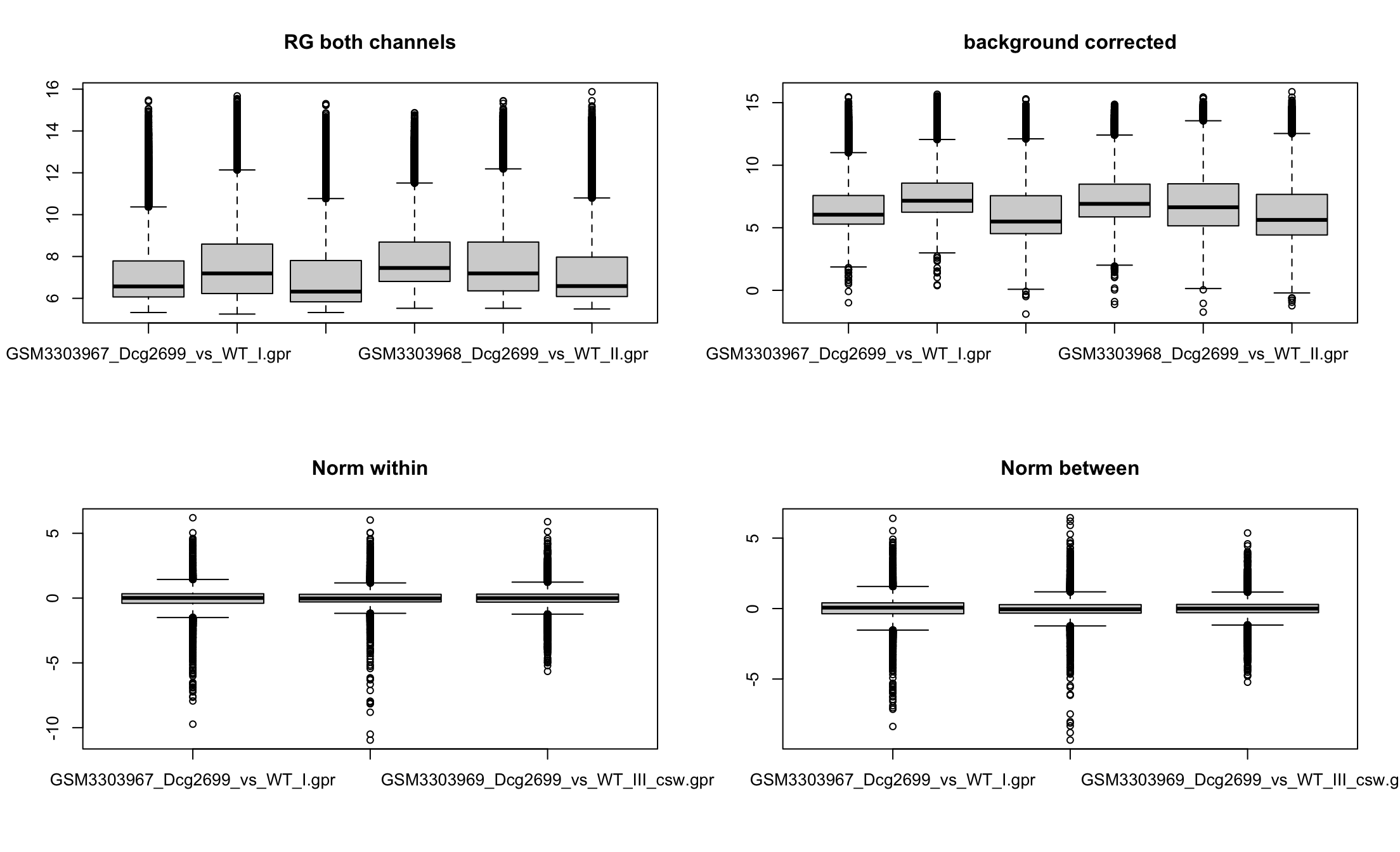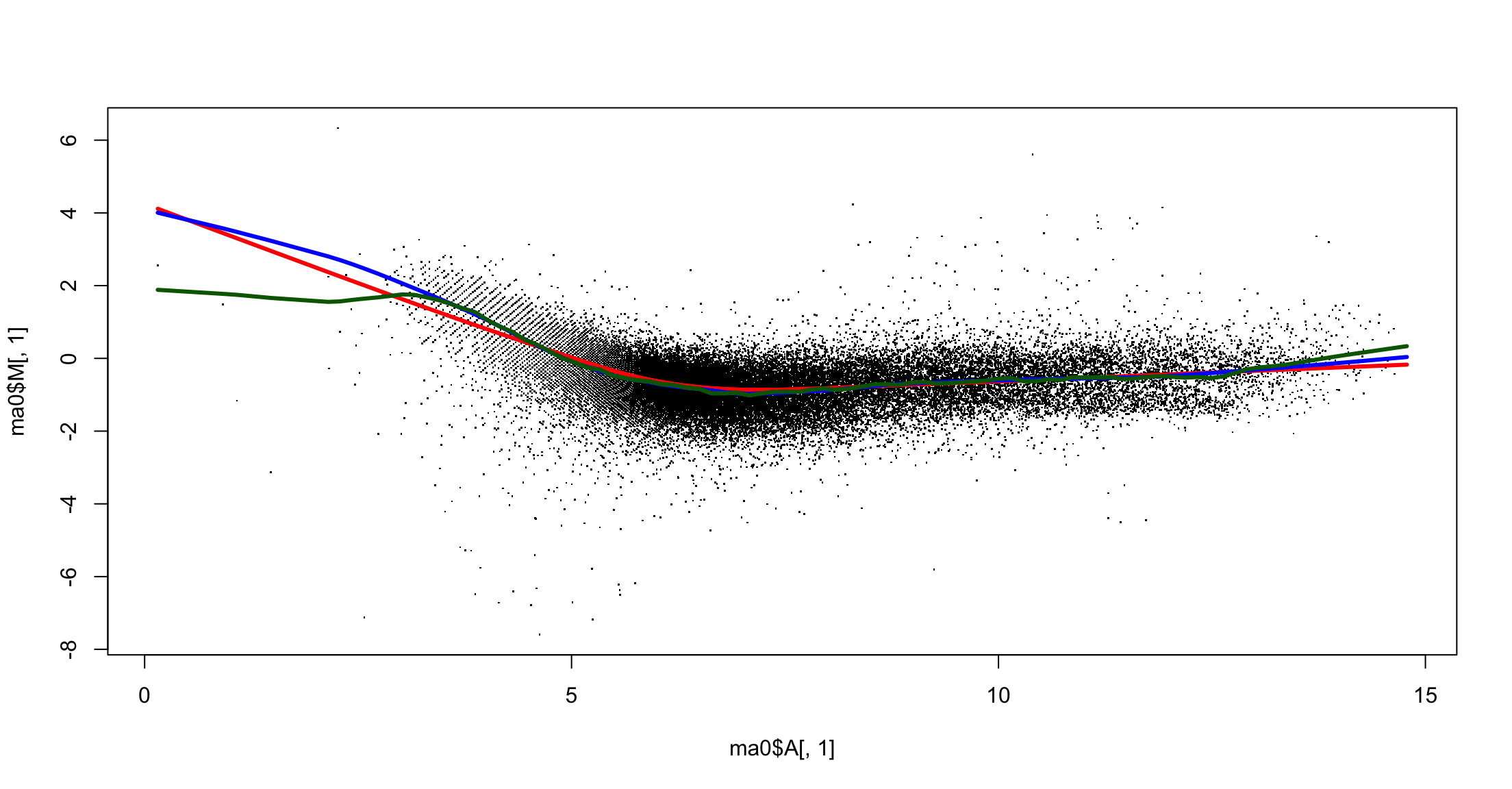# Systems Biology

## Normalization

• Background correction
• Remove technical noise
• Within arrays
• Guarantee that observed differential expression is real
• “Makes average equal to zero”
• Between arrays
• Enables comparison between conditions
• “Makes variances equal”

## Within arrays

• Uses logarithm of war expression
• Remove background signal
• Assumes that some genes are not differentially expressed
• Either housekeeping genes or most of the genes
• they are forced to have zero differential expression

library(limma)
RG <- read.maimages(targets\$Filename, source = "genepix")
Read GSM3303967_Dcg2699_vs_WT_I.gpr.gz
Read GSM3303969_Dcg2699_vs_WT_III_csw.gpr.gz 
RG_corr <- backgroundCorrect(RG, method = "normexp")
Array 1 corrected
Array 2 corrected
Array 3 corrected
Array 1 corrected
Array 2 corrected
Array 3 corrected
MA <- normalizeWithinArrays(RG_corr, method = "loess")
MA.q <- normalizeBetweenArrays(MA, method = "quantile")

## Box plots## Lowess: within array normalization## Pending

• NormExp model for background normalization

• Combining probes for a single gene

• Clustering

• Heathmap

# Single color arrays

## Affymetrix

• Massive arrays

• Hundreds of thousands of spots
• In situ synthesis

• Each oligo has a negative control

## Negative control

• Oligos are short. Let’s say 25 bp

• There may be several oligos for each gene

• Positive match oligos are called $$PM$$

• For each $$PM$$ there is a negative control called $$MM$$

• $$MM$$ oligos have a mismatching basepair in the center bp

• Position 13 if oligos arr 25 bp long

## Normalization

The $$MM$$ probe will not hybridize with the gene, but will hybridize with random fragments

The same random fragments will hybridize with the $$PM$$

The difference $$PM-MM$$ is the signal of the gene